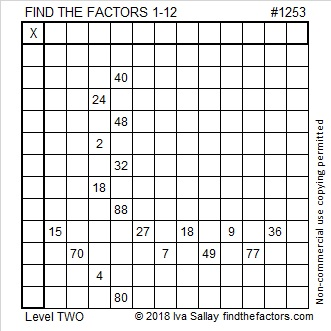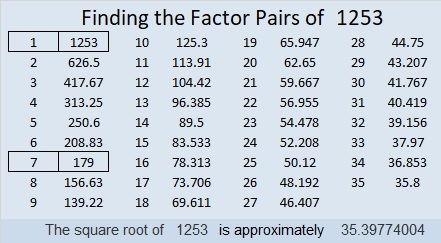# 1253 and Level 2

In what order should the numbers from 1 to 12 be written in the first column and also in the top row so that this puzzle works like a multiplication table?Print the puzzles or type the solution in this excel file: 12 factors 1251-1258

Now I’ll tell you a little bit about the number 1253:

• 1253 is a composite number.
• Prime factorization: 1253 = 7 × 179
• The exponents in the prime factorization are 1 and 1. Adding one to each and multiplying we get (1 + 1)(1 + 1) = 2 × 2 = 4. Therefore 1253 has exactly 4 factors.
• Factors of 1253: 1, 7, 179, 1253
• Factor pairs: 1253 = 1 × 1253 or 7 × 179
• 1253 has no square factors that allow its square root to be simplified. √1253 ≈ 35.397741253 is also the sum of the eleven prime numbers from 89 to 139. Do you know what those prime numbers are?

This site uses Akismet to reduce spam. Learn how your comment data is processed.